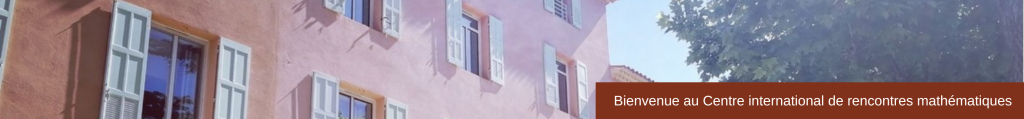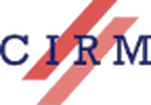SMALL GROUP
Higher Structures in Algebra and Topology
March 9 -12, 2015
 Algebra.  The  theory  of categories has  proven  to be  a valuable algebraic tool to state and to organize the  results  in many  areas in mathematics.  In the  same way,  the  notion  of an operad, which emerged from the study of iterated loop spaces in the early 1970’s, now plays a structural  role by organizing  the many operations with several inputs acting on the various objects in algebra, geometry, topology and mathematical physics. Higher algebra. At the end  of the last century,  it appeared clearly that the introduction  of higher  algebraic notions  was necessary in order  to encode higher structures naturally appearing  in mathematical problems. Homotopy theory  provides  us  with natural phenomena whose description requires higher maps, that is some notion of higher category. In the same way, the algebraic notion of an operad, while already used to describe homotopy algebras, was proved  to be too strict a notion, e.g.  for the purposes of deformation theory. After a long period of research in algebra and topology, the theory of higher structures gave rise recently to radically new and  manageable notions,  like infinity-categories, homotopy  algebras and homotopy operads, which make possible the study of some unsolved questions like formality theorems (Kontsevich)  and cobordism hypothesis (Lurie), for instance. Field theories. In an attempt  to provide well established mathematical definitions to objects studied  in field theory  in physics, several renowned mathematicians introduced the notions of topological  field theories, cohomological field theories and  conformal field theories using categorical or operadic structures on geometrical objects: smooth  manifolds  with boundary (Atiyah), algebraic curves with marked  points  (Kontsevich–Manin), and  Riemann surfaces with holomorphic  holes  (Segal)  respectively. Present project.  This project consists in new foundational developments of high algebra directed  toward the resolution of problems in the topological  study of field theories. It proposes first to enrich one  step further the theory of higher structures with new models, like a  suitable notion of symmetric operad up  to homotopy  and  a  good  notion  of infinity- cooperad. Then  it aims at applying these new methods to the mathematical study of field theories, like En-algebras, factorization algebras, topological field theories, cohomological field theories, vertex algebras and conformal field theories. Organizing Committee Université Nice-Sophia Antipolis) Speakers Bruno Vallette (Université Nice-Sophia Antipolis)Joan Millès (Université Paul Sabatier, Toulouse)Brice Le Grignou (Université Nice-Sophia Antipolis)Eduard Balzin (Université Nice-Sophia Antipolis)Damien Lejay (Institut de Mathématiques de Jussieu – Paris Rive Gauche)Damien Calaque (Université de Montpellier)